Students can Download Maths Chapter 6 Information Processing Ex 6.1 Questions and Answers, Notes Pdf, Samacheer Kalvi 7th Maths Book Solutions Guide Pdf helps you to revise the complete Tamilnadu State Board New Syllabus and score more marks in your examinations.

Tamilnadu Samacheer Kalvi 7th Maths Solutions Term 1 Chapter 6 Information Processing Ex 6.1

Question 1.
A tetromino is a shape obtained by …… squares together.
Solution:
4

Question 2.
Draw a tetromino which passes symmetry ……..
Solution: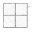Question 3.
Complete the table.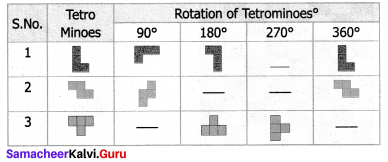Solution: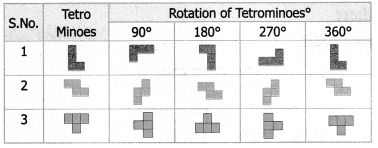Question 4.
Shade the figure completely, by using five tetrominoes shapes only once.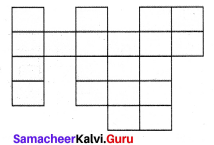Solution: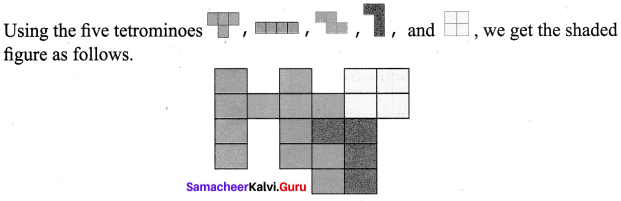Question 5.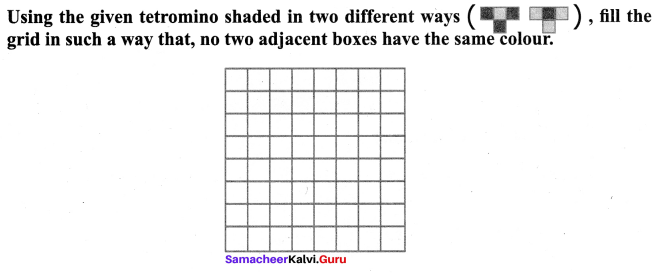Solution: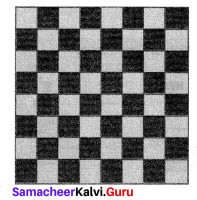More possible ways are theQuestion 6.
Match the tetrominoes of same type.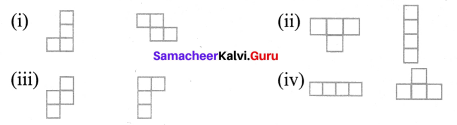Solution: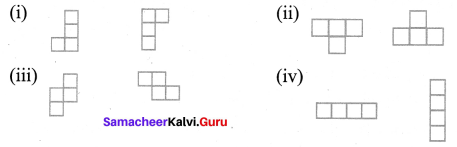Question 7.
Using the given tetrominoes with numbers, compute the 4 × 4 magic square.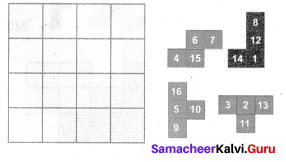Solution: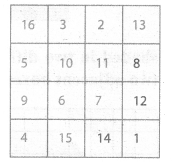(more possible ways are these)